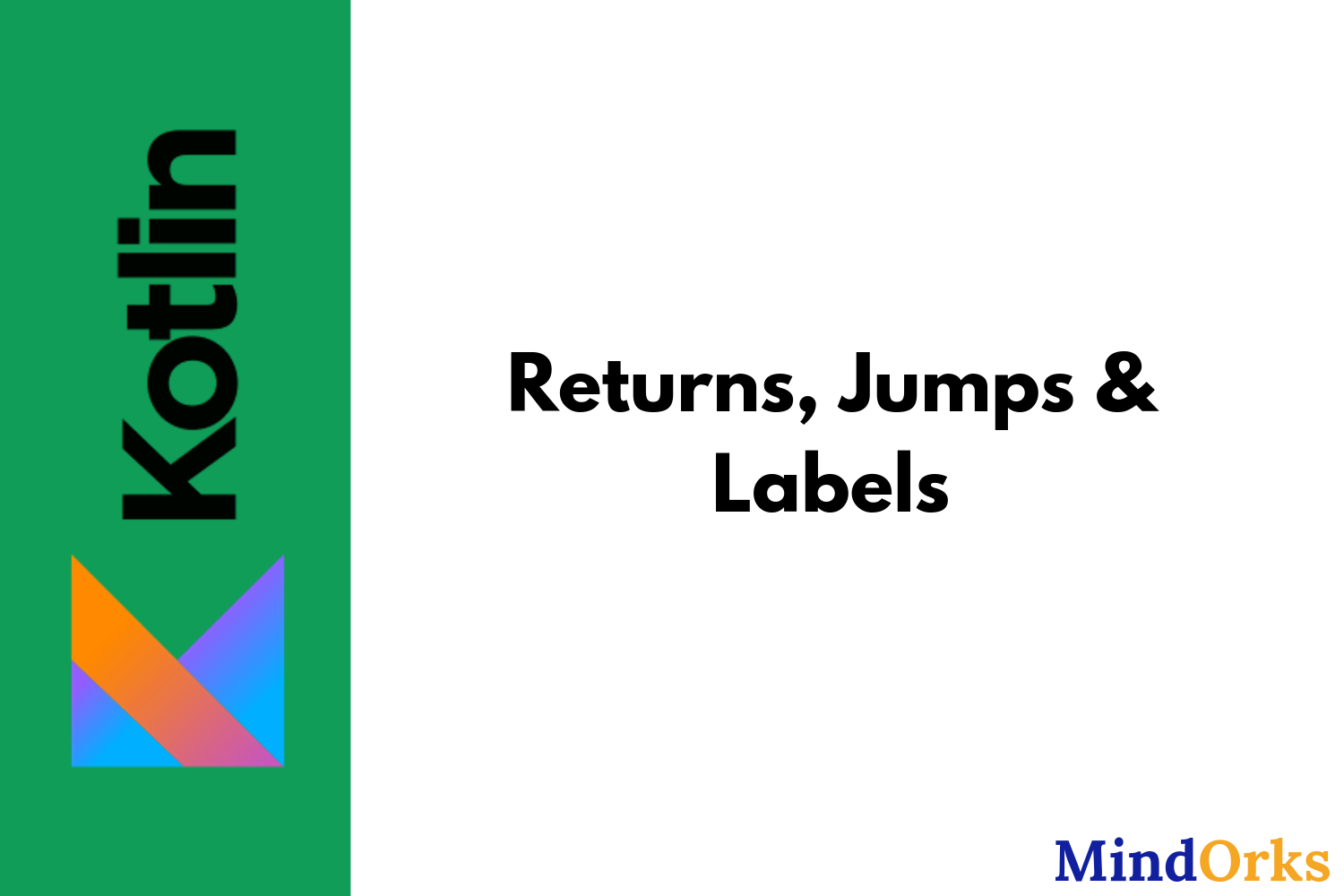# Learn Kotlin — Returns, Jumps & LabelsIn this blog, we will learn about Jumps and Labels in Kotlin. In Kotlin there are three types of Jump expressions i.e.

1. return
2. break
3. continue

So, let’s start by having an intro of labels in Kotlin and then moving towards the Jump expressions.

#### Labels

Any expression written in Kotlin is called labels . For example, if we are having a for-loop in our Kotlin code then we can name that for-loop expression as a label and will use the label name for the for-loop . So, basically we are giving one name to the for-loop and by doing so whenever we want to call the same for-loop , we can just call it by the label name.

We can create a label by using an identifier followed by the “@” sign. For example, name@, loop@, xyz@, etc.

Following is an example of a label:

``````loop@ for (i in 1..10) {
// some code goes here
}``````

Here, in the above example, the name of the for loop is loop@ . Now, we can use the for loop by the label name i.e. by loop@.

#### Jump Expressions

Jump expressions are those expressions that are used to terminate the normal flow of code. For example, if you are executing a 100 line code then if after line number 25 you want to execute line number 57 then it can be done with the help of Jump expressions. As discussed earlier, there are 3 types of Jump expressions i.e. return, break, and continue . Let’s have a look at them.

#### Retur n

The return expression is used to return something from a function body. You can think of a function as a machine and the output of the machine is collected by the return statement.

Without a label, the return statement returns to the nearest enclosing function or anonymous function.

``````fun main(args: Array<String>) {
val myName = name()
println("Name = \$myName")
}

fun name() : String {
return "MindOrks"
}``````

In the above example, the function name() , returns a string having the value as “MindOrks” and this value is stored in the myName variable. So, whenever the function name() is called then the string “MindOrks” will be returned.

While using a labelled return, the control of the program is returned to the specific line in the code. For example, if the length of the code is 100 and the return is at the 45th line then the rest of the code will not be executed.

``````fun foo() {
listOf(1, 2, 3, 4, 5).forEach lit@{
if (it == 3) return@lit // local return to the caller of the lambda, i.e. the forEach loop
print(it)
}
print(" done with explicit label")
}``````

Also, we can return some value from an anonymous function i.e. a function not having any name. The return statement in an anonymous function will return from the anonymous function itself.

``````fun foo() {
listOf(1, 2, 3, 4, 5).forEach(fun(value: Int) {
if (value == 3) return  // local return to the caller of the anonymous fun, i.e. the forEach loop
print(value)
})
print(" done with anonymous function")
}``````

#### Break

A break statement is used to terminate the flow of a loop. If you are not using a label, then the break statement will terminate the nearest enclosing loop i.e. if you are having two nested for-loops and the break statement is present in the inner for-loop then the inner for-loop will be terminated first and after that if another break is added then the outer for-loop will also be terminated.

``````for (i in 1..100) { //loop 1
for (j in 1..100) { //loop 2
if ( j == 10) break
}
}``````

In the above example, if the value of j becomes 10, then loop 2 will be terminated due to the break statement and the execution of loop 1 will continue.

If we are using break with labels then this will terminate the loop marked with that label. For example,

``````loop@ for (i in 1..100) { //loop 1
for (j in 1..100) { // loop 2
if (j == 10) break@loop
}
}``````

In the above code, if the value of j becomes 10, then the outer loop or loop 1 will be terminated and no further loops will be executed.

#### Continue

The continue statement is used to continue or proceed to the next iteration of the loop. For example, if we are having a for-loop of 100 iterations and after some iteration, we used the continue statement, then at that time the rest of the code after the continue statement will not be executed and the next iteration will be started. For example,

``````for (i in 1..100) {
for (j in 1..100) {
if (j == 10) continue
}
}``````

In the above code, when the value of j becomes 10 then no further code will be executed and next iteration will be followed i.e. iteration for j = 11 will be done.

If we are using continue statement with some label, then it will proceed to the next iteration of that particular loop. For example,

``````loop@ for (i in 1..100) { // loop 1
for (j in 1..100) { // loop 2
if (j == 10) continue@loop
}
}``````

In the above code, if the value of j becomes 10, then the next iteration of the outer or the loop 1 will be done because after continue statement the @loop is called.

#### Conclusion

In this blog, we learned about labels in Kotlin. We see how we can assign a name to some loop or statement in Kotlin with the help of labels. We also saw the 3 types of Jump expressions in Kotlin i.e. return, break, and continue.

Hope you learned something new today.